# Artificial neural network

Artificial Neural Networks (ANNs for short) are a connectionist processing model inspired by the architecture of real brains. Artificial neural networks are composed of simple nodes called artificial neurons or Processing Elements (PEs). They can be implemented via hardware (i.e., electronic devices) or software (i.e., computer simulations).

In neural nets, the network behavior is stored in the connections between neurons in values called weights, which represent the strength of each link, equivalent to many components of its biological counterpart.

## Network Components

There are three things to define when creating a neural network:

1. Network architecture: How many neurons the network has, and which neurons are connected to which.
2. Activation function: How a neuron's output depends on its inputs.
3. Learning rule: How the strength of the connections between neurons changes over time.

## An Illustration: The McCulloch-Pitts Neuron

The McCulloch-Pitts neuron precedes modern Neural Nets. It demonstrates that sentential logic can be implemented with a very simple network architecture and activation function, but, because it does not define an activation function, requires hand-tuned weights. It is included here as an illustration.

### M-P Architecture

The basic McCulloch-Pitts network has two input neurons x1 and x2 which fire either 1 or 0 along with a bias neuron xb that always fires 1. These input neurons have weighted directed connections to a single output neuron y. For example, the connection from input neuron x1 to the output neuron y has weight$w_{y,{x_1}}$, and there is no connection at all from output neuron y to x1 (or any of the input neurons). The net input net to output neuron y is the weighted sum of its inputs: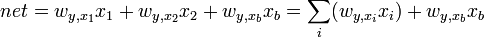$net = w_{y,{x_1}} x_1 + w_{y,{x_2}} x_2 + w_{y,{x_b}} x_b = \sum_i ( w_{y,{x_i}} x_i) + w_{y,{x_b}} x_b$

Because the bias neuron always fires 1:$net = \sum_i ( w_{y,{x_i}} x_i) + w_{y,{x_b}} \cdot 1 = \sum_i ( w_{y,{x_i}} x_i) + w_{y,{x_b}}$

### M-P Activation Function

The activation function of the M-P neuron is the step function of its net input: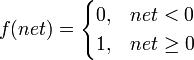$f(net) = \begin{cases} 0, & net < 0\\ 1, & net \geq 0 \end{cases}$

### Illustrations

To demonstrate how fiddling with the network weights allows us to change the logical function implemented by the network, we next select specific weights and see how the network behavior changes.

#### Illustration 1: Implementing AND

We will select a set of weights to define a network whose output fires 1 if both inputs are firing 1, and where the output fires 0 otherwise. Let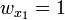$w_{x_1} = 1$,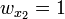$w_{x_2} = 1$, and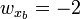$w_{x_b} = -2$.

Consider first the case where both inputs are 1. Then we have:$net = \sum_i ( w_{y,{x_i}} ) + w_{y,{x_b}} = 1 \cdot 1 + 1 \cdot 1 + (-2) = 0$

So by definition of our activation function, we have f(net) = 1.

Consider next the case where only one input is 1. Then we have:$net = \sum_i ( w_{y,{x_i}} ) + w_{y,{x_b}} = 0 \cdot 1 + 1 \cdot 1 + (-2) = -1$

And by definition of our activation function, we have f(net) = 0. Finally, consider the case where neither input is 1: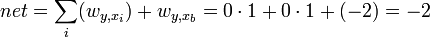$net = \sum_i ( w_{y,{x_i}} ) + w_{y,{x_b}} = 0 \cdot 1 + 0 \cdot 1 + (-2) = -2$

And by definition of our activation function, we have f(net) = 0. So this network fires 1 if and only if both input neurons are firing 1.

#### Illustration 2: Implementing OR

We will select a set of weights to define a network whose output fires 1 if either or both inputs are firing 1, and where the output fires 0 otherwise. Let$w_{x_1} = 1$,$w_{x_2} = 1$, and$w_{x_b} = -1$.

Consider first the case where both inputs are 1. Then we have:$net = \sum_i ( w_{y,{x_i}} ) + w_{y,{x_b}} = 1 \cdot 1 + 1 \cdot 1 + (-1) = 1$

So by definition of our activation function, we have f(net) = 1.

Consider next the case where only one input is 1. Then we have: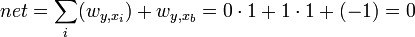$net = \sum_i ( w_{y,{x_i}} ) + w_{y,{x_b}} = 0 \cdot 1 + 1 \cdot 1 + (-1) = 0$

And by definition of our activation function, we have f(net) = 1. Finally, consider the case where neither input is 1: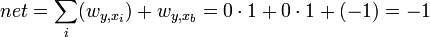$net = \sum_i ( w_{y,{x_i}} ) + w_{y,{x_b}} = 0 \cdot 1 + 0 \cdot 1 + (-1) = -1$

And by definition of our activation function, we have f(net) = 0. So this network fires 1 if either or both of its neurons are firing 1.Some content on this page may previously have appeared on Citizendium.